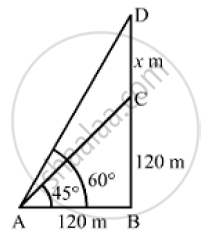# The Angle of Elevation of the Top of a Tower at a Distance of 120 M from a Point a on the Ground is 45 .Find the Height of the Flagstaff [Use Sqrt(3) 1.732] - Mathematics

The angle of elevation of the top of a tower at a distance of 120 m from a point A on the ground is 45 . If the angle of elevation of the top of a flagstaff fixed at the top of the tower, at A is 60 , then find the height of the flagstaff [Use sqrt(3) 1.732]

#### SolutionLet BC and CD be the heights of the tower and the flagstaff, respectively.
We have,
AB  = 120m,  ∠BAC = 45º ,∠BAD = 60°
Let CD = x
In  ΔABC,

tan 45° =  (BC)/(AB)

⇒ 1 =(BC)/120

⇒  BC = 120m

Now , in   ΔABD,

 tan 60º (BD )/(AB)

⇒ sqrt(3)= (BC +CD)/ 120

⇒ BC + CD = 120 sqrt(3)

⇒ x = 120 sqrt( 3 )-120

⇒ x = 120 ( sqrt(3)-1)

⇒ x = 120 ( 1.732 -1)

⇒  x = 120 (0.732)

⇒ x = 87.84 ~~ 87.8m

So, the height of the flagstaff is 87. 8 m.

Concept: Heights and Distances
Is there an error in this question or solution?
Chapter 14: Height and Distance - Exercises

#### APPEARS IN

RS Aggarwal Secondary School Class 10 Maths
Chapter 14 Height and Distance
Exercises | Q 5

Share